Courses

# Worksheet Question - Exponents and Powers Class 7 Notes | EduRev

## Mathematics (Maths) Class 7

Created by: Praveen Kumar

## Class 7 : Worksheet Question - Exponents and Powers Class 7 Notes | EduRev

The document Worksheet Question - Exponents and Powers Class 7 Notes | EduRev is a part of the Class 7 Course Mathematics (Maths) Class 7.
All you need of Class 7 at this link: Class 7

Q.1. Fill in the blanks :

(a)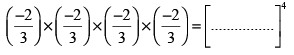(b) (–3)3 × (–3)4 = ....................

Ans.

(a)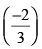(b) (–3)7

Q.2. Evaluate :

(a) Find the value of x :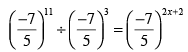(b) Find the value of a :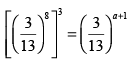Ans. (a) x = 3

(b) a = 23

Q.3. Match of column :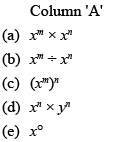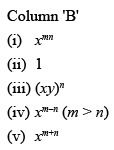Ans.

Column 'A'               (Column 'B'

(a)                            (v)
(b)                            (iv)
(c)                             (i)
(d)                            (iii)
(e)                            (ii)

Q.4. Write in the standard form :
(a) The distance between Earth and Moon is 384,000 km.
(b) Speed of light in vacuum is 300,000,000 m/s
(c) 0.0034256

Ans. (a) 3.84 × 105 km
(b) 3 × 108 m/s
(c) 3.4256 × 10–3

Q.5. Find the value of x :

(a)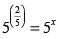(b)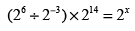Ans. (a) 32
(b) 23

Q.6. Simplify :

(a)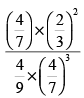(b)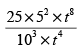Ans.

(a)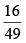(b)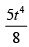Q.7. Simplify :

(a) (–3)2 × (–5)2

(b) [(-16)6 ÷ (-16)3 ] x (-16)3

Ans. (a) 225

(b) 1

Q.8. Find the value of :
(a) 2° × 3° × 4°
(b) 3° × 5° + 19°
(c) (7° ÷ 3°) × (8° – 5°)
(d) 4° × 6° + 100°

Ans.

(a) 1

(b) 3

(c) 0

(d) 2

Q.9. Fill in the blanks :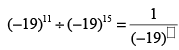Ans.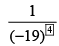Q.10. Simplify and write the answer in scientific rotation : (a) (5 × 103) × (3 × 105

(b)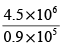Ans. (a) 1.5 ×109
(b) 5 × 10

Q.11. Find m for the following :

(a)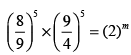(b)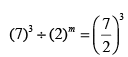Ans. (a) m = 5
(b) m = 3

Q.12. Using the standard form, write number 73984 in expanded form.

Ans. 7 × 104 + 3 ×10+ 9 × 10+ 8 × 101 + 4 × 10°

Offer running on EduRev: Apply code STAYHOME200 to get INR 200 off on our premium plan EduRev Infinity!

,

,

,

,

,

,

,

,

,

,

,

,

,

,

,

,

,

,

,

,

,

;